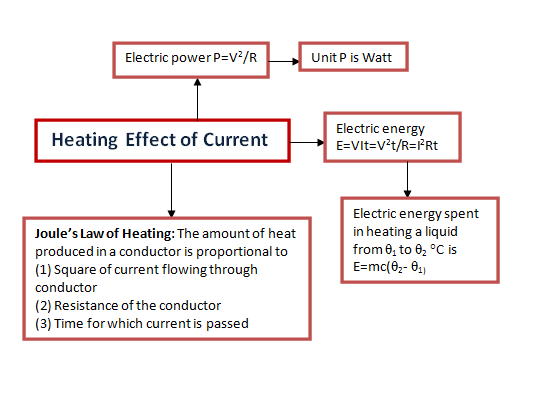# Thermal Effect of Current

## Introduction

• We have already learned the electric current and the physics behind it in the previous chapter
• We have also discussed the mechanism of flow of current in a conductor but not the physical consequences of flow of electric current are related to other forms of energy
• In this chapter we will study about causes and consequences of electric current
• In the nutshell ,what we will study in this chapter is the connection between the electricity and thermal energy.

## (2) Heating effect of current

• In previous chapter while discussing electric energy and power ,we learned that IΔV amount of energy is lost per second when a current I flows through a potential ΔV and this energy appears in the form of heat energy
• Due to the conversion of electric energy into heat energy the conductor becomes hot .This effect is known as Joule's Heating and this heating is thermodynamics irreversible. Cause Behind Joule's Heating:-

• Explanation behind the Joule's heating is that when a potential difference ΔV is maintained between the ends of a conductor,the free electrons in the conductor are accelerated towards the higher potential end of the conductor
• In their way electrons frequently collided with the positive ions of the conductor due to which their velocity decreased
• This the energy electrons gained on account of acceleration is transferred to the positive lattice ions or atoms and electrons then returns to their equilibrium distribution of velocities
• Thus ,lattice ions receives energy randomly at the average rate of IΔV per unit time
• Ions spends this energy by vibrating about their mean positions resulting in rise in the temperature of the conductor
• This way Joule's heating nothing but the conversion of electrical energy into heat energy

Concept Map